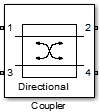# Coupler

Model ideal frequency-independent couplers with S-parameters

• Library:
• RF Blockset / Circuit Envelope / Junctions

•## Description

The Coupler block models four port directional couplers in a circuit envelope environment as an ideal S-parameter model. The four ports of the coupler are `Input port` (Port 1), `Through port` (Port 2), `Isolated port` (Port 3), `Coupled port` (Port 4).

Directional couplers are used to sample forward and reflected waves propagating along a transmission line. Directional couplers find uses in many RF design applications such as line power sensors and transmitter automatic level controls.

Hybrid couplers are used to split or combine signals with specific phase relations.

## Parameters

expand all

Coupler type, specified as:

• `Directional coupler`

The default option is `Directional coupler`. The S-parameters matrix for the `Directional coupler` is:

$\left[\begin{array}{cccc}{r}_{l}& {i}_{l}& {i}_{s}& c\\ {i}_{l}& {r}_{l}& c& {i}_{s}\\ {i}_{s}& c& {r}_{l}& {i}_{l}\\ c& {i}_{s}& {i}_{l}& {r}_{l}\end{array}\right]$

where:

• rl = 10(-ReturnLoss/20)

• il = j10(-InsertionLoss/20)

• is = j10(-(Coupling+Directivity)/20)

• c = 10(-Coupling/20)

Note

In release 2019a, the S-parameters representation of the Directional Coupler block is altered to permit a valid implementation for all specified mask values. This implementation adds a 90 degree phase shift to s12, s13, s21, s24, s31, s34, s42, and s43 terms. In addition, a -180 phase shift is added to the s14, s23, s32 and s41 terms.

Use this option to model coupler parameters from data sheets.

• `Coupler symmetrical`

The S-parameters matrix for the `Coupler symmetrical` is:

$\left[\begin{array}{cccc}0& \alpha & 0& j\beta \\ \alpha & 0& j\beta & 0\\ 0& j\beta & 0& \alpha \\ j\beta & 0& \alpha & 0\end{array}\right]$

where:

• |α| ≤ 1 = Power transmission coefficient

• β = sqrt(1– α*α)

• `Coupler antisymmetrical`

The S-parameters matrix for the `Coupler antisymmetrical` is:

$\left[\begin{array}{cccc}0& \alpha & 0& \beta \\ \alpha & 0& -\beta & 0\\ 0& -\beta & 0& \alpha \\ \beta & 0& \alpha & 0\end{array}\right]$

where:

• |α| ≤ 1 = Power transmission coefficient.

• β = sqrt (1– α*α)

• `Hybrid quadrature (90deg)`

The S-parameters matrix for the `Hybrid quadrature(90deg)` is:

$\left[\begin{array}{cccc}0& -j/\sqrt{2}& 0& -1/\sqrt{2}\\ -j/\sqrt{2}& 0& -1/\sqrt{2}& 0\\ 0& -1/\sqrt{2}& 0& -j/\sqrt{2}\\ -1/\sqrt{2}& 0& -j/\sqrt{2}& 0\end{array}\right]\text{ }$

• `Hybrid rat-race`

The S-parameters matrix for the `Hybrid rat-race` is:

$\left[\begin{array}{cccc}0& -j/\sqrt{2}& 0& -j/\sqrt{2}\\ -j/\sqrt{2}& 0& j/\sqrt{2}& 0\\ 0& j/\sqrt{2}& 0& -j/\sqrt{2}\\ -j/\sqrt{2}& 0& -j/\sqrt{2}& 0\end{array}\right]\text{ }$

• `Magic tee`

The S-parameters matrix for the ```Magic tee``` is:

$\frac{\left[\begin{array}{cccc}0& 0& 1& 1\\ 0& 0& 1& -1\\ 1& 1& 0& 0\\ 1& -1& 0& 0\end{array}\right]}{\sqrt{2}}$

The Divider block uses the `ispassive` function to test the passivity of the S-parameters matrix.

Fraction of input signal power coupled to output port of the ```Directional coupler```, specified as a nonnegative and real scalar. The default value is `0` dB.

#### Dependencies

To enable this parameter, select `Directional coupler` in Select component tab.

Ratio of power at coupled port to power at isolated port of the `Directional coupler`, specified as a nonnegative and real scalar. The default value is `inf`.

#### Dependencies

To enable this parameter, select `Directional coupler` in Select component tab.

Loss of signal power between input and output ports of the ```Directional coupler```, specified as a nonnegative and real scalar. The default value is `inf`.

#### Dependencies

To enable this parameter, select `Directional coupler` in Select component tab.

Loss of signal power due to impedance mismatch of the ```Directional coupler```, specified as a nonnegative, and real scalar. The default value is `inf`.

#### Dependencies

To enable this parameter, select `Directional coupler` in Select component tab.

Transmitted signal power of the `Directional coupler`, specified as a real scalar. The default value is 0.

#### Dependencies

To enable this parameter, select ```Coupler symmetrical``` or ```Coupler antisymmetrical``` in Select component tab.

Reference impedance of coupler, specified as a scalar or three-tuple. The default value is `50 Ohms`.

Select this parameter to ground and hide the negative terminals. To expose the negative terminals, clear this parameter. By exposing these terminals, you can connect them to other parts of your model.

By default, this option is selected.

## Version History

Introduced in R2014a